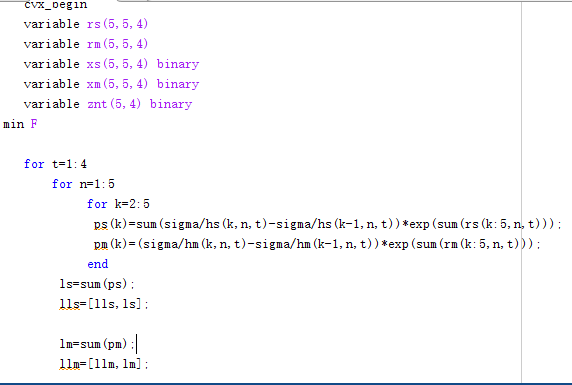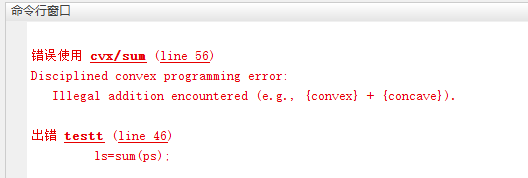# How to represent thisexpression in CVX

This is a mixedinteger convex programming (MICP). I want to know how the sum in the optimization problem is represented, and should my optimization variable be set to a matrix? I hope you can give me some advice, thank you very much！

the xknt is binary variable.

You can declare something such as:
variable r(I,N,T)
or whatever the appropriate dimensions are. Then it’s just a matter of getting the indices correct for the summations, for which you can use sum, the same as it would be in “regular” MATLAB Of course this presumes that the \sigma^2 difference terms evaluate to nonnegative.

I write the left-hand side of 4b and 4c as followsBut it doesn’t workCould you give me some suggestions for modification. Thank you very much!

A model that has nonlinear equalities cannot be convex.

In this case, those are assignments, not constraints. So there are no nonlinear equalities.

I believe the cause of the error must be that not all of the sum of \sigma^2 difference terms are nonnegative. Hence those elements of ps are concave. And some of those sum of \sigma^2 difference terms are positive, which makes those elements of ps convex. You will need all those terms to be convex. Note the statement in my previous post “Of course this presumes that the \sigma^2 difference terms evaluate to nonnegative.”.

So you will need to change the input data so that all of the sum of \sigma^2 difference terms are nonnegative.

Thank you very much! I solved it!